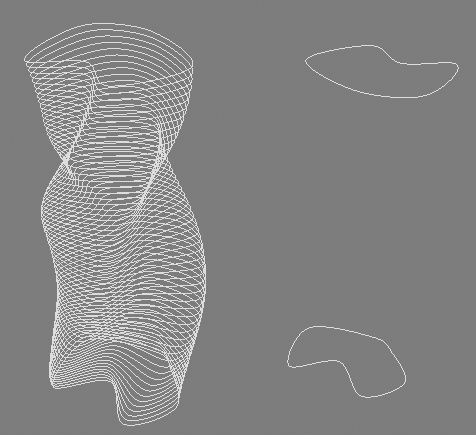# Lines Sets(Lines Set_02)

In this chapter we change also the positions of the lines themselves during the vertices positions changing gradually..

s_array = \$ as array
m = 60
for s = 1 to (s_array.count - 1) do
(
s1 = s_array[s]
s2 = s_array[s + 1]
d = distance s1 s2
h = d/ma
n = numknots s1
for i = 0 to m do
(
myshape = copy s1
for j = 1 to n do
(
p = getknotpoint s2 1 j
q = getknotpoint s1 1 j
v = p - q
d = distance p q
setknotpoint myshape 1 j (q + (i as float)/m * v)
)
centerpivot myshape
if s != 1 do dis = (distance s_array s_array[s])
if s == 1 do dis = 0

myshape.pos = [10 * cos(10 * i),10 * sin(10 * i),(h * i) + dis]
r = eulerangles 0 0 (i * 3)
rotate myshape r

)
)
redrawviews()### Very cool stuff guys.

Very cool stuff guys.

John Martini
Digital Artist
http://www.JokerMartini.com (new site)### new approach

```fn filterLine s = ((isKindOf s SplineShape or isKindOf s Line) and s.modifiers.count == 0)
fn smokeLines height:300 profile: cnt: rnd:false c1:(color 20 20 20) c2:(color 255 255 255) =
(
if (filterLine profile != true) then #abort else
(
if rnd == false do (local stepR = ((c2.r - c1.r)/cnt), stepG = ((c2.g - c1.g)/cnt), stepB = ((c2.b - c1.b)/cnt))
local h = height/cnt, gkp = getknotpoint, skp = setknotpoint, \
numS = (numSplines profile), splArr = #()
for c = 0 to cnt do
(
local newProfile = copy profile ; append splArr newProfile
for s = 1 to numS do
(
local numK = (numknots profile s)
for k = 1 to numK do
(
local kPos = gkp profile s k
skp newProfile s k (kPos + (c/2 as float)/cnt * height)
)
)

newProfile.render_sides = 6 ; newProfile.render_threshold = 60

newProfile.pos = point3 (cos(c)) (sin(c)) (h*c)
rotate newProfile (eulerangles 0 0 (c*2))
)
for l in 1 to splArr.count do
(
if rnd == true then (splArr[l].wirecolor = random [0,0,0] [255,255,255])
else splArr[l].wirecolor = (point3 ((stepR*l)+c1.r-stepR) ((stepG*l)+c1.r-stepG) ((stepB*l)+c1.r-stepB))
)
redrawviews() ; select splArr
)
)
smokeLines profile:\$Line001 cnt:50```

barigazy### Yeah, very good man... but

Yeah, very good man... but how to make the transition from one line to another?

kimarotta.com.br
3d Artist